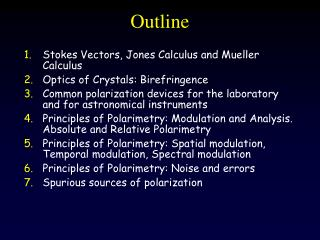DownloadDownload PresentationOutline

# Outline

Download Presentation## Outline

- - - - - - - - - - - - - - - - - - - - - - - - - - - E N D - - - - - - - - - - - - - - - - - - - - - - - - - - -
##### Presentation Transcript

1. Outline • Stokes Vectors, Jones Calculus and Mueller Calculus • Optics of Crystals: Birefringence • Common polarization devices for the laboratory and for astronomical instruments • Principles of Polarimetry: Modulation and Analysis. Absolute and Relative Polarimetry • Principles of Polarimetry: Spatial modulation, Temporal modulation, Spectral modulation • Principles of Polarimetry: Noise and errors • Spurious sources of polarization

2. A. López Ariste Stokes Vector, Jones Calculus,Mueller Calculus playing around with matrices

3. Assumptions: • A plane transverse electromagnetic wave • Quasi-monochromatic • Propagating in a well defined direction z

4. Jones Vector

5. Jones Vector: It is actually a complex vector with 3 free parameters It transforms under the Pauli matrices. It is a spinor

6. The Jones matrix of an optical device In group theory: SL(2,C)

7. From the quantum-mechanical point of view, the wave function cannot be measured directly. Observables are made of quadratic forms of the wave function: J is a density matrix : The coherence matrix

8. Like Jones matrices, J also belongs to the SL(2,C) group, and can be decomposed in the basis of the Pauli matrices. Is the Stokes Vector

9. The Stokes vector is the quadractic form of a spinor. It is a bi-spinor, or also a 4-vector

10. 4-vectors live in a Minkowsky space with metric (+,-,-,-)

11. The Minkowski space I Partially polarized light Cone of (fully polarized) light Fully polarized light V Q

12. M is the Mueller matrix of the transformation

13. From group theory, the Mueller matrix belongs to a group of transformations which is the square of SL(2,C) Actually a subgroup of this general group called O+(3,1) or Lorentz group

14. The cone of (fully polarized) light I Lorentz boost = de/polarizer, attenuators, dichroism V Q

15. The cone of (fully polarized) light I 3-d rotation = retardance, optical rotation V Q

16. Mueller Calculus • Any macroscopic optical device that transforms one input Stokes vector to an output Stokes vector can be written as a Mueller matrix • Lorentz group is a group under matrix multiplication: A sequence of optical devices has as Mueller matrix the product of the individual matrices

17. Mueller Calculus: 3 basic operations • Absorption of one component • Retardance of one component respect to the other • Rotation of the reference system

18. Mueller Calculus: 3 basic operations • Absorption of one component

19. Mueller Calculus: 3 basic operations • Absorption of one component • Retardance of one component respect to the other

20. Mueller Calculus: 3 basic operations • Absorption of one component • Retardance of one component respect to the other • Rotation of the reference system

21. A. López Ariste Optics of Crystals: Birefringence

22. Chapter XIV, Born & Wolf

23. !!

24. Ellipsoïd

25. Ellipsoïd

26. Three types of crystals A spherical wavefront

27. Three types of crystals • Two apparent waves propagating at different speeds: • An ordinary wave, with a spherical wavefront propagating • at ordinary speed vo • An extraordinary wave with an elliptical wavefront, its speed • depends on direction with characteristic values vo and ve

28. Three types of crystals

29. z s The ellipsoïd of D in uniaxial crystals De The two propagating waves are linearly polarized and orthogonal one to each other Do

30. Typical birefringences • Quartz +0.009 • Calcite -0.172 • Rutile +0.287 • Lithium Niobate -0.085

31. A. López Ariste Common polarization devices for the laboratory and for astronomical instruments

32. Linear Polarizer

33. Retarder

34. Savart Plate

35. Glan-Taylor Polarizer • Glan-Taylor.jpg

36. Glan-Thompson Polarizing Beam-Splitter

37. Rochon Polarizing Beamsplitter